# Difference between revisions of "Dynamic programming"

Dynamic programming (DP) is a technique for solving problems that involves computing the solution to a large problem using previously-computed solutions to smaller problems. DP, however, differs from recursion in that in DP, one solves the smallest possible subproblems (the base cases) first, and works up to the original problem, storing the solution to each subproblem in a table (usually a simple array or equivalent data structure) as it is computed so that its value can be quickly looked up when computing the solutions to larger subproblems. Most problems that are solved using DP are either counting problems or optimization problems, but there are others that fall into neither category. Because DP is a scheme for solving problems rather than a specific algorithm, its applicability is extremely broad, and so a number of examples are presented in this article to help explain exactly what DP is and what it can be used for.

By some estimates, nearly a third of all problems that appear on the IOI and national olympiads and team selection competitions are intended to be solved using DP. This may have decreased in modern years, since competitors are getting better and better at solving DP problems. (The high incidence of DP problems on the Canadian Computing Competition, for one, will become evident in the examples below.)

## Basic principles of DP

Not every optimization problem can be solved using DP, just as not every optimization problem can be solved using recursion. In order for an optimization problem to be solvable using DP, it must have the property of optimal substructure. And most of the time, when DP is useful, the problem will exhibit overlapping subproblems. (We can solve problems using DP even when they do not exhibit overlapping subproblems; but such problems can also easily be solved using naive recursive methods.) We will examine the problem Plentiful Paths from the 1996 Woburn Challenge and show how it exhibits both properties.

### Optimal substructure

An optimization problem is said to have optimal substructure when it can be broken down into subproblems and the optimal solution to the original problem can be computed from the optimal solutions of the subproblems. Another way of saying this is that the optimal solution to a large problem contains an optimal solution (sometimes more than one) for a subproblem (maybe more than one). This is often stated and proved in its contrapositive form, which is that if a solution to a problem instance contains any suboptimal solutions to subinstances, then the solution is not optimal for the original instance. Let's put this into the context of Plentiful Paths.

The Plentiful Paths problem asks us to optimize the number of apples collected in travelling from the lower-left square to the upper-right square. We start at (1,1) and end up eventually at (M,N). It is fairly clear that at some point we must pass through either (M-1,N) or (M,N-1). (Think about this carefully if you don't see why this is.) Suppose that the optimal solution involved travelling through (M-1,N). So we take some path to reach (M-1,N) and then we take one step to reach the end, (M,N). A bit of thought should convince you that we must collect as many apples as possible on the path from (1,1) to (M-1,N) if we want to collect the maximum possible number of apples on the path from (1,1) to (M,N). This is because if we found a better path to get to (M-1,N) - that is, one with more apples along the way - then we would also collect more apples in total getting to (M,N).

Having said that, the optimal path to (M-1,N) must itself consist of an optimal path to one of the squares immediately before it, for the same reason: either (M-2,N) or (M-1,N-1)... and so on.

(Notice that if A is a subproblem of B, and B is a subproblem of C, then A is a subproblem of C as well. Then, a DP solution would first solve A, then use this to solve B, and finally use that to solve C. But if A is a subproblem of B, B cannot be a subproblem of A. This is because, in order to find an optimal solution to B, we would first need an optimal solution to A, but to find an optimal solution to A, we would first need an optimal solution to B, so ultimately we can solve neither problem.)

The Plentiful Paths problem has optimal substructure because any optimal solution to an instance of Plentiful Paths contains an optimal solution to a subproblem. In general, in a DP solution, we start by solving the smallest possible subproblems (such as: "how many apples can we collect going from (1,1) to (1,1)?") and work our way up to larger problems.

### Overlapping subproblems

A problem is said to have overlapping subproblems when some of its subproblems are repeated. For example, in Plentiful Paths, in order to find an optimal path to (M,N), we must find the optimal paths to (M-1,N) and (M,N-1). To find the optimal path to (M-1,N), we need to find the optimal paths to both (M-2,N) and (M-1,N-1); to find the optimal path to (M,N-1) we must find the optimal path to both (M-1,N-1) and (M,N-2). Notice that the subproblem (M-1,N-1) was repeated. It is the fact that subproblems are repeated that gives DP its massive efficiency advantage over the recursive solution in the next section.

### Example: recursive solution to Plentiful Paths

Based on the optimal substructure property of the Plentiful Paths problem, we might write the following pseudocode:

function plentiful_paths(A,x,y)
if x=1
if y=1 then
return A[x,y]
else
return A[x,y]+plentiful_paths(A,x,y-1)
else
if y=1 then
return A[x,y]+plentiful_paths(A,x-1,y)
else
return A[x,y]+max(plentiful_paths(A,x-1,y),plentiful_paths(A,x,y-1))
input M,N,A
print plentiful_paths(A,M,N)


Here, A is a matrix (two-dimensional array) in which an entry is 1 if the corresponding position in the grid contains an apple and 0 if it does not contain an apple; the function plentiful_paths(A,x,y) returns the maximum number of apples that can be retrieved in going from (1,1) to (x,y) in grid A.

• If x and y are both 1, then the question is how many apples we can collect if we travel from (1,1) to (1,1); this will simply be 1 if there is an apple at (1,1) and 0 otherwise. (That is the quantity A[x,y].)
• If x is 1 but y is not, then in order to travel from (1,1) to (x,y), we must first travel from (1,1) to (x,y-1), then take one step to reach (x,y). The maximum number of apples we can collect in travelling to (x,y) is equal to the maximum number of apples we can collect in travelling to (x,y-1) (this is plentiful_paths(A,x,y-1)), plus one if there is an apple at (x,y).
• Similarly, if x is not 1 and y is 1, then we collect the maximum number of apples in travelling to (x-1,y), then we add one apple if there is an apple in the square (x,y).

If x and y are both greater than 1, then the optimal path to (x,y) goes through either (x-1,y) or (x,y-1). The maximum number of apples collected in reaching (x,y) will be either the maximum number of apples collected in reaching (x-1,y) or the same for (x,y-1), whichever is greater (that is to say, max(plentiful_paths(A,x-1,y),plentiful_paths(A,x,y-1))), plus one if there is an apple at (x,y).

The problem with this recursive solution is that, even though the value of plentiful_paths(A,i,j) will be the same every time it is calculated as long as A, i, and j stay the same, it might calculate that value hundreds of times. For example, in calculating plentiful_paths(A,5,5), we will actually calculate plentiful_paths(A,1,1) seventy times. This is because of the overlapping subproblems. For larger grids, we waste so much time recalculating values that the algorithm becomes impractically slow and therefore unusable. We can avoid this by using memoization. However, DP presents an alternative solution.

(Note that in a real recursive solution we would not pass A by value, since its value never changes and thus passing by value would slow down the solution massively by copying A over and over again. Instead, A would be either passed by reference or stored as a global variable. In the latter case, it would disappear from the parameter list altogether.)

### Example: dynamic solution to Plentiful Paths

input M,N,A
for x = 1 to M
for y = 1 to N
if x=1 then
if y=1 then
dp[x,y]=A[x,y]
else
dp[x,y]=A[x,y]+dp[x,y-1]
else
if y=1 then
dp[x,y]=A[x,y]+dp[x-1,y]
else
dp[x,y]=A[x,y]+max(dp[x-1,y],dp[x,y-1])
print dp[M,N]


In the dynamic solution, dp[x,y] represents the maximum possible number of apples that can be obtained on some path from (1,1) to (x,y). As soon as we calculate this value, we store it in the two-dimensional array dp[]. We see that this solution follows essentially the same logic as the recursive solution, and our final answer is dp[M,N]. However, no entry in the dp[] table is calculated more than once. When M and N are each 1000, it would take far longer than the age of the universe for the recursive solution to finish executing even if the entire surface of the Earth were covered in transistors being used exclusively to execute the algorithm, but the dynamic solution would run in under a second on a modern desktop computer.

This solution exemplifies both the optimal substructure property (because in calculating dp[x,y], an optimal solution for the square (x,y) we potentially examine the values of dp[x-1,y] and dp[x,y-1], optimal solutions for possible preceding squares, or subproblems) and the overlapping subproblems property (most entries in the dp[] table will be examined twice, but only calculated once). It is the presence of overlapping subproblems that gives DP such a huge efficiency advantage over the naive recursive solution: we will only calculate the solution to each subproblem once, and from then on we will look it up whenever it is needed. Finally, we have computed the solution bottom-up, by solving the smallest subproblems first so that by the time we reach a particular subproblem, we have already computed the solutions to any subproblems on which that subproblem depends. Contrast this with the recursive solution, which considers the largest subproblem (the original problem) first; it is top-down.

## Optimization example: Change problem

Dynamic change is the name given to a well-known algorithm for determining how to make change for a given amount of money using the fewest possible coins, assuming that we have an unlimited supply of coins of every denomination. For example, if the available denominations are 1 cent, 5 cents, 10 cents, and 25 cents, then we can make change for 48 cents by using one 25-cent coin, two 10-cent coins, two 5-cent coins, and three 1-cent coins, that is, 8 coins in total. There are other ways to make change for the same amount (for example, four 10-cent coins and eight 1-cent coins), but they all use more than 8 coins. Other sets of denominations are not so easy; for example, suppose we have at our disposal 17-cent, 14-cent, 9-cent, and 7-cent coins. It is now not immediately obvious how best to make change for a dollar (The solution is to use four 17-cent coins, one 14-cent coin, and two 9-cent coins.) Nor is it immediately obvious that it is impossible to make change for 29 cents.

The problem Golf from the 2000 Canadian Computing Competition is exactly analogous. Here, the distance to the hole is the amount for which we wish to make change, and each golf club is a denomination with value equal to how far it will hit the golf ball.

The general form of this problem is: given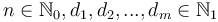$n \in \mathbb{N}_0, d_1, d_2, ..., d_m \in \mathbb{N}_1$, find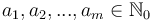$a_1, a_2, ..., a_m \in \mathbb{N}_0$ such that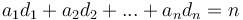$a_1 d_1 + a_2 d_2 + ... + a_n d_n = n$ and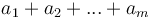$a_1 + a_2 + ... + a_m$ is minimal.$n$ corresponds to the amount we wish to change, each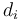$d_i$ to a denomination, and each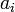$a_i$ to the number of coins of a given denomination we wish to use.

This problem exhibits optimal substructure in the following sense. Suppose we have found out how to make change for$n$ cents using some minimal collection of coins, and we take one coin away (with value$x$), leaving a collection of coins with total value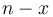$n-x$. Then, what we are left with must be an optimal way to make change for$n-x$. This is because if we had any better way (fewer coins) of making change for$n-x$, then we could just add the coin of value$x$ back in, and get a better way of making change for$n$ cents than what we originally assumed was minimal, a contradiction.

For example, if we have 48 cents in the form of one 25-cent coin, two 10-cent coins, two 5-cent coins, and three 1-cent coins, and we take away the 25-cent coin, then we are left with two 10-cent coins, two 5-cent coins, and three 1-cent coins, that is, 7 coins totalling 23 cents. However, 7 coins is not the least number of coins required to change 23 cents; we could do it in 5 (using two 10-cent coins and three 1-cent coins). This proves that our original way of making change for 48 cents was not optimal, as we can just add the quarter back in (so we'll have one 25-cent coin, two 10-cent coins, and three 1-cent coins, or 6 coins totalling 25 cents) and get a better way. Whenever we have a suboptimal solution to a subinstance, we will also have a suboptimal solution to the original instance; so an optimal solution to the original instance is only allowed to contain optimal solutions to subinstances.

To solve the change problem using DP, we start by considering the simplest possible case: when the amount to be changed is zero. Then, the optimal (and only) solution is to use no coins at all. But whenever we change a positive amount, we must use at least one coin. If we take any coin (with value$x$) away from the optimal change for amount$n$, then we are left with optimal change for$n-x$. This implies that we can make optimal change for$n$ using at least one of the following ways: make optimal change for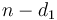$n-d_1$ and add one coin of value$d_1$; or make optimal change for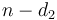$n-d_2$ and add one coin of value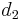$d_2$; ...; or make optimal change for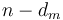$n-d_m$ and add one coin of value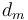$d_m$. This is because if none of these are optimal, then when we make optimal change for$n$, and remove some coin of value$d_i$, leaving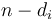$n-d_i$, we will have optimal change for$n-d_i$, but we already know that adding a coin of value$d_i$ to the leftover collection does not give optimal change for$n$, and yet it regenerates the original collection, which we assumed to be optimal, and this is a contradiction.

We conclude that to make optimal change for$n$, we consider all denominations with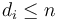$d_i \leq n$; for each of these, we figure out how many coins are required to make change for$n-d_i$, and then add one to make change for$n$; and after considering all these possibilities, the one that uses the fewest total coins is the optimal way to make change for$n$ coins. (Note that if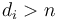$d_i > n$, then there is simply no way that any way of making change for$n$ will use the coin with value$d_i$ at all, so we don't even need to consider it.)

Also, if it is not possible to make change for$n - d_i$ no matter what$i$ is (in other words, subtracting any denomination is guaranteed to give something unchangeable), then we can give up; there is clearly no way to make change for$n$ either.

Pseudocode (prints either the minimum number of coins required to make change, or concludes that it is impossible):

input n, m, array d
c ← 0    // can make change for 0 using 0 coins
for i ∈ [1..n]
c[i] ← ∞
for j ∈ [1..m]
if d[j] ≤ c[i]
c[i] ← min(c[i], c[i-d[j]]+1)
if c[n] = ∞
print Impossible
else
print c[n]


## Counting example: Keep on Truckin'

The problem Keep on Truckin' from the 2007 Canadian Computing Competition is easily expressible in an abstract form. A number of points are marked on a horizontal line, with x-coordinates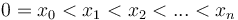$0 = x_0 < x_1 < x_2 < ... < x_n$. We start out at the leftmost point and we wish to reach the rightmost point. To do so, we take a sequence of steps. In each step, we walk from our current point to some point to our right. The length of a step must be at least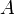$A$, but not more than$B$. We wish to know in how many different ways we can complete the trip. Two ways of completing the trip are considered different if one of them visits a point that the other does not.

### Disjoint and exhaustive substructure

This problem is a counting problem, not an optimization problem. Counting problems cannot exhibit optimal substructure, because they are not optimization problems. Instead, the kinds of counting problems that are amenable to DP solutions exhibit a different kind of substructure, which we shall term disjoint and exhaustive substructure. (Counting problems do, however, often exhibit overlapping subproblems, just like optimization problems.)

In this particular problem, to count the number of ways to get from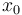$x_0$ to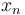$x_n$, we observe that we can categorize all possible paths according to the last step taken. That is, we either get from$x_0$ to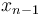$x_{n-1}$ and then take one additional step from$x_{n-1}$ to$x_n$, or we get from$x_0$ to$x_{n-2}$ and then take one additional step from$x_{n-2}$ to$x_n$, or we get from$x_0$ to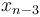$x_{n-3}$ and then take one additional step from$x_{n-3}$ to$x_n$, and so on. The categories are disjoint in the sense that two paths from different categories are never the same path, since two paths cannot possibly be the same if their last steps are not the same. They are exhaustive in the sense that every possible path from$x_0$ to$x_n$ will end up in one of the categories (in particular, if its last step is from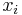$x_i$ to$x_n$, then it ends up in the same category as all the other paths whose last step is from$x_i$ to$x_n$).

Having made this observation—and categorized all the paths—we can now count the total number of paths. Since the categories are disjoint and exhaustive, we simply add the number of paths in each category to obtain the total number of paths. But each category can be represented as a subproblem. The number of ways to go from$x_0$ to$x_n$ with the last step being from$x_i$ to$x_n$ is the same as the number of ways to go from$x_0$ to$x_i$, period.

So we can finally conclude that the total number of ways to go from$x_0$ to$x_n$ is the sum of the number of ways to go from$x_0$ to$x_i$ for all$i$ such that we can make the trip from$x_i$ to$x_n$ in a single step (that is,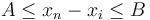$A \leq x_n - x_i \leq B$).

### Pseudocode implementation

input n, sorted sequence x[], A, B
dp ← 1                    // one way to get from x_0 to x_0, namely, by not taking any steps at all
for i ∈ [1..n]
dp[i] ← 0
j = i-1
while x[i] - x[j] < A    // x_j is too close
j = j - 1
while x[i] - x[j] ≥ B
dp[i] = dp[i] + dp[j]
j = j - 1
print dp[n]